# Precious metals

In 2006-2009, the value of precious metals changed rapidly. The data in the following table represent the total rate of return (in percentage) for platinum, gold, an silver from 2006 through 2009:

Year Platinum Gold Silver
2009 62.7 25.0 56.8
2008 -41.3 4.3 -26.9
2007 36.9 31.9 14.4
2006 15.9 23.2 46.1

a. Compute the geometric mean rate of return per year for platinum, gold, and silver from 2006 through 2009.

b. What conclusions can you reach concerning the geometric mean rates of the three precious metals?

Result

r1 =  1.11
r2 =  1.206
r3 =  1.176

#### Solution:Leave us a comment of example and its solution (i.e. if it is still somewhat unclear...):

Showing 1 comment:Dr Math
So all metals have approx the same rate of price growth per year over time#### To solve this verbal math problem are needed these knowledge from mathematics:

Looking for a statistical calculator? Our percentage calculator will help you quickly calculate various typical tasks with percentages.

## Next similar examples:

1. Value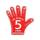Find the value of the expression: 6!·10^-3
2. Theorem proveWe want to prove the sentence: If the natural number n is divisible by six, then n is divisible by three. From what assumption we started?
3. Tenth memberCalculate the tenth member of geometric sequence when given: a1=1/2 and q=2
4. Powers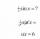Is true for any number a,b,c equality:? ?
5. A perineumA perineum string is 10% shorter than its original string. The first string is 24, what is the 9th string or term?
6. GP - 8 itemsDetermine the first eight members of a geometric progression if a9=512, q=2
7. The city 2Today lives 167000 citizens in city. How many citizens can we expect in 11 years if their annual increase is 1%?
8. LogarithmDetermine the number whose decimal logarithm is -3.8.
9. Five membersWrite first 5 members geometric sequence and determine whether it is increasing or decreasing: a1 = 3 q = -2
10. Investment1000\$ is invested at 10% compound interest. What factor is the capital multiplied by each year? How much will be there after n=12 years?
11. Im>0?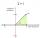Is -10i a positive number?
12. Virus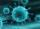We have a virus that lives one hour. Every half hour produce two child viruses. What will be the living population of the virus after 3.5 hours?
13. Geometric sequence 4It is given geometric sequence a3 = 7 and a12 = 3. Calculate s23 (= sum of the first 23 members of the sequence).
14. Binomials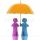To binomial ? add a number to the resulting trinomial be square of binomial.
15. The city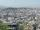At the end of 2010 the city had 248000 residents. The population increased by 2.5% each year. What is the population at the end of 2013?
16. Radioactive materialA radioactive material loses 10% of its mass each year. What proportion will be left there after n=6 years?
17. Annual pensionCalculate the amount of money generating an annual pension of EUR 1000, payable at the end of the year and for a period of 10 years, shall be inserted into the bank to account with an annual interest rate of 2%